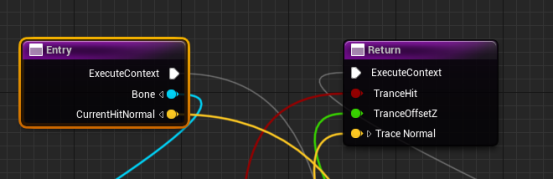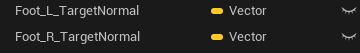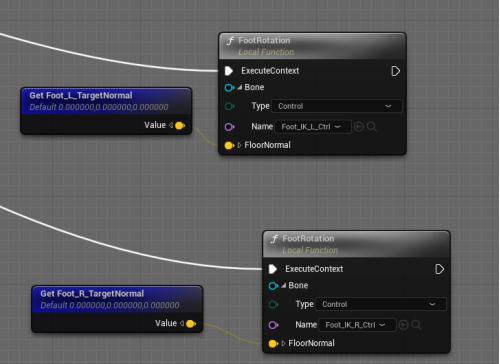top of page
Search
• Liling Liu

# [Control Rig] Unreal Engine 5.2 Control Rig 教學 - Foot IK - Leg Alignment - # 6 ｜腿部對齊 ｜Tutorial

Leg AlignmentFirst, go back to the Foot Trace Function that was created at the beginning to get the Hit Normal information.

Foot TraceSpring Interpolate is used to smoothly move the current to Target.Final Foot Trace FunctionConnect function

LeftRightConnect togetherCreate Function: Foot Rotation

Foot Offset

Input add: Bone (Rig Element Key), FloorNormal(Vector)Use Aim Math for rotation, and treat the Hit Normal value as the Target of Aim Math. Primary - Axis : X=0, Y=0, Z=1。Secondary - Axis: X=0, Y=0, Z=1, Target: X=1, Y=0, Z=0

Kind: DirectionUse the bone/controller Rotation value to multiply the Aim Math Rotation value and set it back to the bone/controller Rotation value.Final Foot Rotation functionConnect function

Left/RightFinal Result2 views
bottom of page